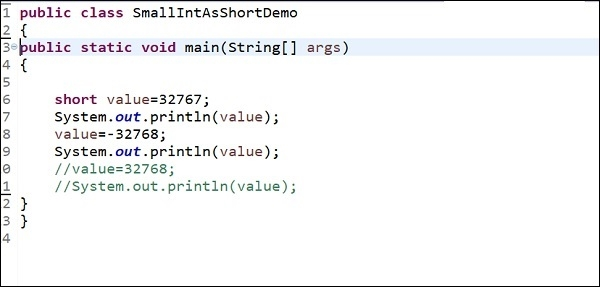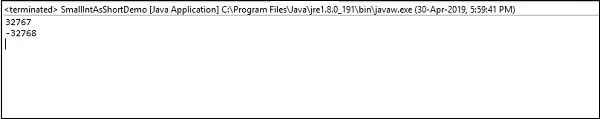# What is the Java equivalent to MySQL's smallint?

The short is equivalent to MySQL’s small int. The Java short takes 2 bytes that has the range -32768 to 32767 while MySQL smallint also take 2 bytes with same range.

Here is the demo code of short in Java −

public class SmallIntAsShortDemo {
public static void main(String[] args) {
short value = 32767;
System.out.println(value);
value = -32768;
System.out.println(value);
// value = 32768;
// System.out.println(value);
}
}

The snapshot is as follows −This will produce the following output −

32767
-32768

Here is the snapshot of the output we ran in EclipseIDE −The MySQL smallint takes 2 bytes with same range.

Advertisements